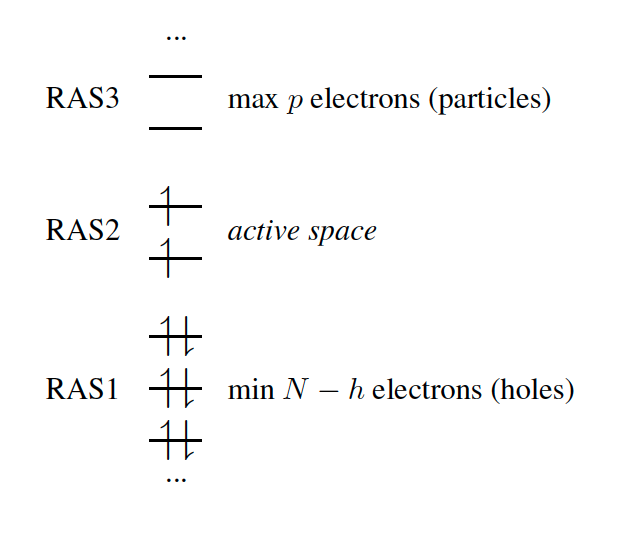Searching....

# 7.12.2 The Restricted Active Space (RAS) Scheme

(July 14, 2022)

In the RAS formalism, one divides the orbital space into three subspaces called RAS1, RAS2 and RAS3 (Fig. 7.2). The RAS-CI states are defined by the number of orbitals and the restrictions in each subspace.Figure 7.2: Orbital subspaces in RAS-CI employing a ROHF triplet reference.

The single reference RAS-CI electronic wave functions are obtained by applying a spin-flipping or excitation operator $\hat{R}$ on the reference determinant $|\phi^{\text{(0)}}\rangle$.

 $\left|{\Psi}^{\text{RAS}}\right>=\hat{R}\left|\phi^{\text{(0)}}\right>$ (7.110)

The $\hat{R}$ operator must obey the restrictions imposed in the subspaces RAS1, RAS2 and RAS3, and can be decomposed as:

 $\hat{R}=\hat{r}^{\text{RAS2}}+\hat{r}^{h}+\hat{r}^{p}+\hat{r}^{hp}+\hat{r}^{2h% }+\hat{r}^{2p}+...$ (7.111)

where $\hat{r}^{\text{RAS2}}$ contains all possible electronic promotions within the RAS2 space, that is, a reduced full CI, and the rest of the terms generate configurations with different number of holes ($h$ super-index) in RAS1 and electrons in RAS3 ($p$ super-index). The current implementation truncates this series up to the inclusion of hole and particle contributions, i.e., the first three terms on the right hand side of Eq. (7.111).